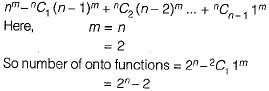Courses

# Set Theory & Algebra - 2

## 15 Questions MCQ Test GATE Electrical Engineering (EE) 2022 Mock Test Series | Set Theory & Algebra - 2

Description
This mock test of Set Theory & Algebra - 2 for GATE helps you for every GATE entrance exam. This contains 15 Multiple Choice Questions for GATE Set Theory & Algebra - 2 (mcq) to study with solutions a complete question bank. The solved questions answers in this Set Theory & Algebra - 2 quiz give you a good mix of easy questions and tough questions. GATE students definitely take this Set Theory & Algebra - 2 exercise for a better result in the exam. You can find other Set Theory & Algebra - 2 extra questions, long questions & short questions for GATE on EduRev as well by searching above.
QUESTION: 1

### Some group (G, o) is known to be abelian. Then, which one of the following is true for G?

Solution: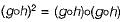Since group is abelian so it is commutative as well as associative.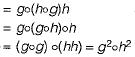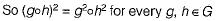QUESTION: 2

### Let R be a sym m etric and transitive relation on a set A Then

Solution:

A relation which is symmetric and transitive, need not foe reflexive relation.
(i) R = {}; on the set A = {a, b}. The relation R is symmetric and transitive but not reflexive.
(ii) R = {(a, a), (b, b)}; on the set A = {a, b}.
The relation R is symmetric, transitive and also reflexive.
∴ A relation is transitive and symmetric relation but need not be reflexive relation.

QUESTION: 3

### Let A and B be sets and let Ac and Bc denote the complements of the sets A and B. The set (A - B) ∪ (B - A) ∪ (A ∩ B) is equal to

Solution: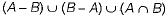Representing above set using Venn diagram as follows: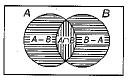∴ By Venn diagram,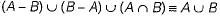QUESTION: 4

Which one of the following is false?

Solution:

In option (c), the set of all strings over a finite alphabet ∑ doesn’t forms a group under concatenation because the inverse of a string doesn’t exist with respect to concatenation.

QUESTION: 5

The binary relation R = {(1, 1), (2, 1), (2, 2), (2, 3), (2, 4), (3, 1), (3, 2), (3, 3), (3, 4)},on the set A = {1 , 2 , 3, 4} is

Solution:

The relation R doesn’t contain (4, 4), so R is not reflexive relation.
Since relation R contains ( 1 ,1 ) , (2, 2) and (3, 3).
Therefore, relation R is also not irreflexive.
That R is transitive, can be checked by systematically checking for all (a, b) and (b, c) in R, whether (a, c) also exists in R.

QUESTION: 6

Let' P(S) denote the power set of a set S. Which of the following is always true?

Solution:

φ always present in any powerset of a set and φ is the only common element between P(S) and P(P(S)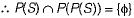QUESTION: 7

Consider the set ∑* of all strings over the alphabet ∑ = {0,1}. ∑* with the concatenation operator for strings

Solution: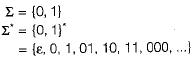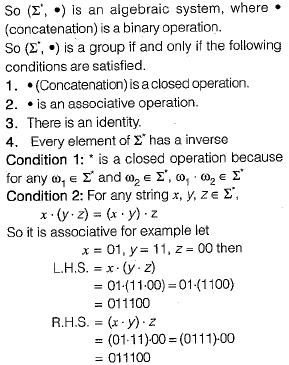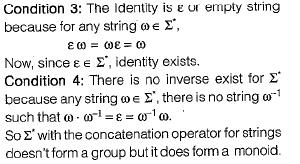QUESTION: 8

Consider the binary relation:
S = {(x, y) | y = x + 1 and x, y ∈ {0, 1, 2, ...}}
The reflexive transitive closure of S is

Solution: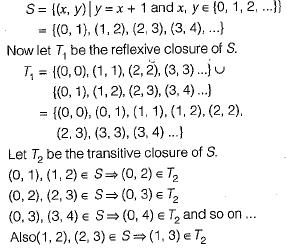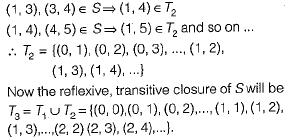QUESTION: 9

In a class of 200 students, 125 students have taken Programming Language course, 85 students have taken Data Structures course, 65 students have taken Computer Organization course; 50 students have taken both Programming Language and Data Structures, 35 students have taken both Data Structures and Computer Organization; 30 students have taken both Data Structures and Computer Organization, 15 students have taken all the three courses. How many students have not taken any of the three courses?

Solution: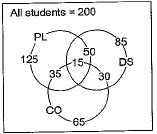According to inclusion - exclusion formula: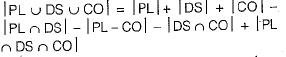PL = students who have taken programming.
DS = Students who have taken Data structures.
CO = Students who have taken Computer Organization.
So, the number of students who have taken atleast 1 of the 3 courses is given by:
= 125 + 85 + 65 - 50 - 35 - 30 + 15
= 175
Therefore, the number of students who have not taken any of the 3 courses is
= Total students-students taken atleast 1 course
= 200-175 = 25

QUESTION: 10

Let A, B and C be non-empty sets and let X = (A - B ) -C and Y = (A - C) - (B - C)  Which one of the following is TRUE?

Solution: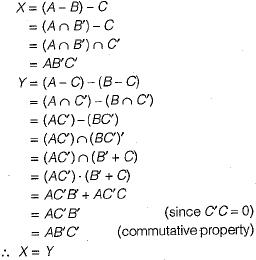QUESTION: 11

The set {1, 2, 4, 7, 8,11,13,14} is a group under multiplication modulo 15. The inverses of 4 and 7 are respectively

Solution: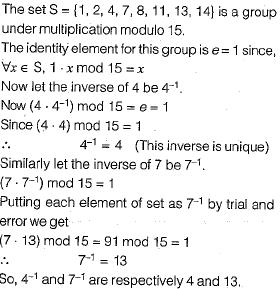QUESTION: 12

A relation R fs defined on ordered pairs of integers as follows: (x, y) R (u, v) if x < u and y > v. Then R is

Solution: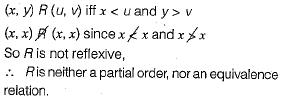QUESTION: 13

Let P, Q and R be sets let A denote the symmetric difference operator defined as PΔG = ( P ∪ Q) - (P ∩ Q). Using Venn diagrams, determine which of the following is/are TRUE?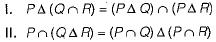Solution:

P Δ Q = PQ' + PQ
where Δ is symmetric difference between P and Q.
1.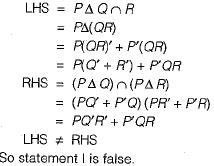2.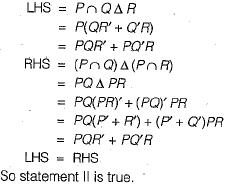QUESTION: 14

Consider the binary relation: R = {(x, y), (x, z), (z, x), (z, y)} on the set {x, y, z}. Which one of the following is TRUE?

Solution: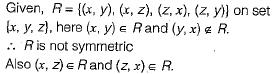∴ R is not antisymmetric.
R is neither-symmetric nor anti-symmetric.

QUESTION: 15

How many onto (or surjective) functions are there from an n-element (n ≥ 2) set to a 2-element set?

Solution:

Let n = 2. There are only 2 onto functions as shown below: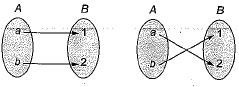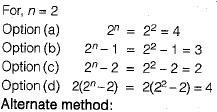The number of onto functions from a set A with m elements to set B with n elements where n < m is given by